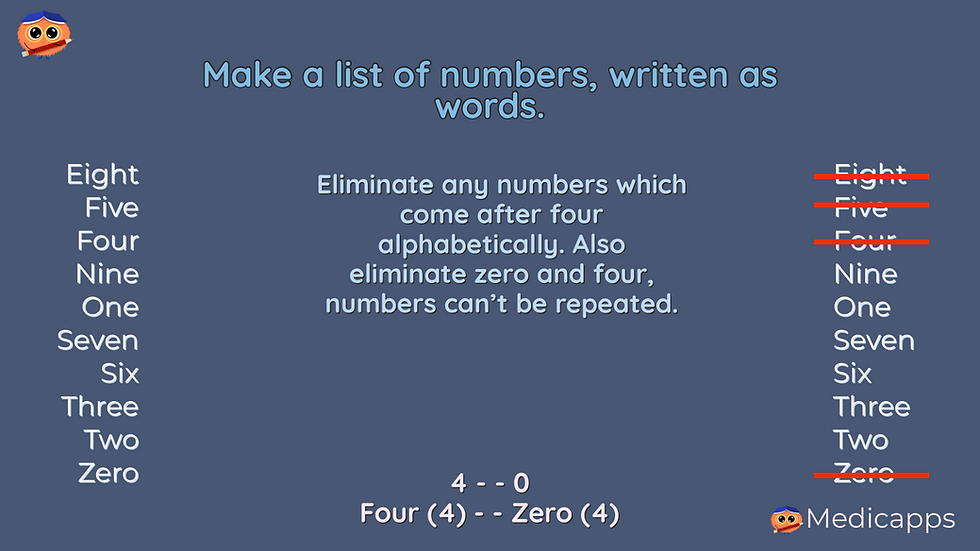top of page
Search

# BMAT Problem solving: Part two

Updated: Mar 16

Problem solving is all about finding and collating the information in the question and then working through it in a logical way. Finally double check the question before you select your answer, because easy mistakes are often in the multiple choice answers.Here is a worked example of a problem solving question from the BMAT, showing how to work through the information step by step. This example question is. A little more time consuming than previous examples. To learn this, write down the question now and after reading this post, try to work through it without the instructions. Proving it to yourself is the best way to learn how to approach these puzzles. The second best thing is practice!Strategy:

1. Find and sort the information in the question.

2. Use clues to eliminate answers

3. Use trial and error logically to resolve the missing digits

Clues from the question:

The numbers are arranged in alphabetical order.

The sum of the numbers is equal to the number of letters in the words.

The first number is four and the last number is zero.

No number is repeated.

The numbers we have are 4 - - 0 where the dashes denote the unknown numbers.

First write down a list of all the numbers spelled out.

The question says the numbers are arranged in alphabetical order, so any number which come before the letter four in the alphabet can be excluded. No number can be repeated, so four and zero can also be excluded. The only purpose of this information, is to allow us to exclude several answers.The new list looks like this, where the number in brackets is the number of letters in the word.)

nine (4)

one (3)

seven (5)

six (3)

three (5)

two (3)The question tells us:

‘When the digits are written as words… the total number of the letters is the same as the numerical sum of the digits themselves.’After eliminating as many possibilities as we can, it comes to trial an error. Here to save time and get the right answer, it is important to work through the options in a methodical manner, not just make random guesses.

Half of the numbers left have three letters, so there is a high probability that one of these will be part of the answer. Writing a column for possible combinations, the sum of numbers and the sum of letters, will help you see as soon as you come across the correct answer, next to the numbers written as words and with the number of letters in the word next to it.

Note: As we have already used the clue about the numbers being in alphabetical order, it is of no further use, the numbers and letters will add up the same way, what ever order they are in.

Note, many candidates fall at the last hurdle where it asks you, not for the pin numbers themselves. but the sum of just the missing numbers. This is a common mistake that you should try to avoid, the multiple choice answers usually include these easy mistakes.

In this case the pin is 4290, the missing digits are 2 and 9 and the addition of these is 11, The correct answer therefore is F.

It is practice that makes perfect and you will get better and better at working through these puzzles in a logical way, the more you try. It can be slow and clunky to start, but its faster and more accurate once you have practiced it. So try this question and then practice others.

Good luck!